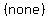Find the standard form of the equation of the ellipse satisfying the given condi

User Generated

Qnja46550

Mathematics

Description

Find the standard form of the equation of the ellipse satisfying the given conditions.

Endpoints of major axis: (7, 9) and (7, 3)
Endpoints of minor axis: (5, 6) and (9, 6)

A. (x - 7)2/6 + (y - 6)2/7 = 1

B. (x - 7)2/5 + (y - 6)2/6 = 1User generated content is uploaded by users for the purposes of learning and should be used following Studypool's honor code & terms of service.So the equationbecomesorAnonymous
Excellent! Definitely coming back for more study materials.Studypool4.7Trustpilot4.5Sitejabber4.4